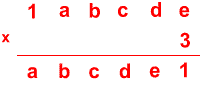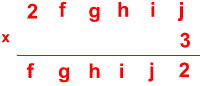#### You may also like### Pebbles

Place four pebbles on the sand in the form of a square. Keep adding as few pebbles as necessary to double the area. How many extra pebbles are added each time?### Bracelets

Investigate the different shaped bracelets you could make from 18 different spherical beads. How do they compare if you use 24 beads?### Sweets in a Box

How many different shaped boxes can you design for 36 sweets in one layer? Can you arrange the sweets so that no sweets of the same colour are next to each other in any direction?

# Trebling

##### Age 7 to 11Challenge LevelCan you replace the letters with numbers?
Is there only one solution in each case?

Once you've had a chance to think about it, click below to see how two different pupils began working on the task.

Here is Abdullah's work:

"For each problem I first looked to find a number that would make the ones column accurate, then I substituted the number for the answer in the tens column and then continued the process until the calculation was complete."

Joshua wrote:

"I wrote out single digit multiples of three up to 9 because each letter was one digit. I noticed that the numbers 1 to 9 only appeared once in the ones column of the answers. I looked at the question and realised that 3 x e had to be 21 because it was the only answer ending in 1. This meant that e had to be 7.

I carried the 2 and took it from 7 (the other e) and got 5. So d x 3 had to end in 5 which meant d had to be 5 because 5 x 3 = 15. I then repeated the process."

Can you take each of these starting ideas and develop it into a solution?